Question

Two fair six-sided dice are rolled. What is the probability that one die shows exactly three more than the other die (for example, rolling a 1 and 4, or rolling a 6 and a 3)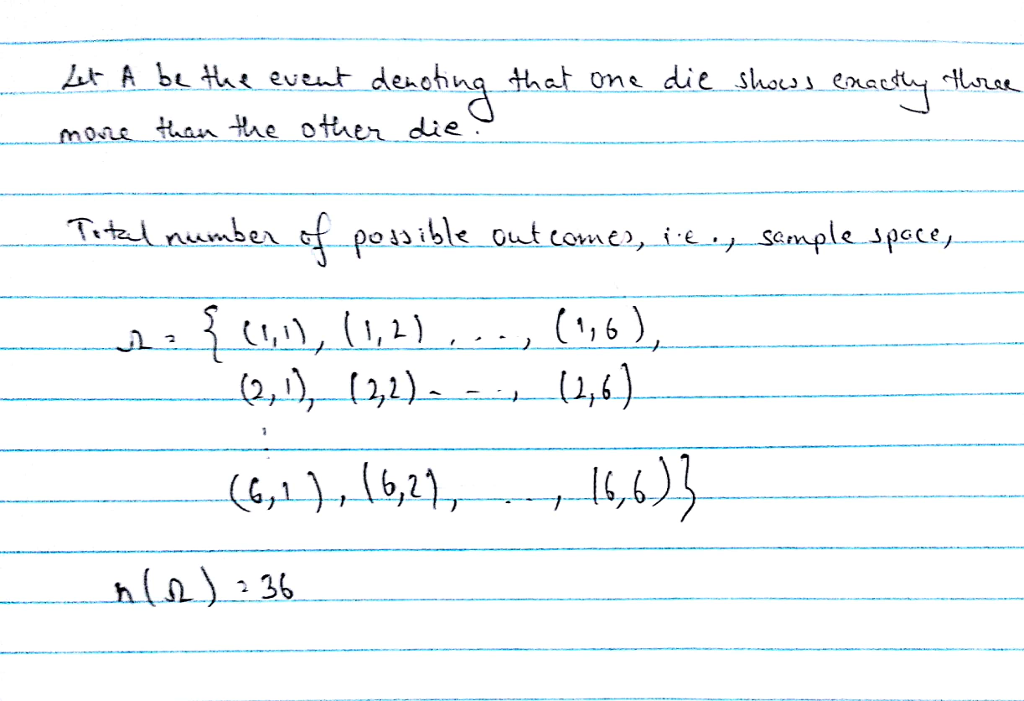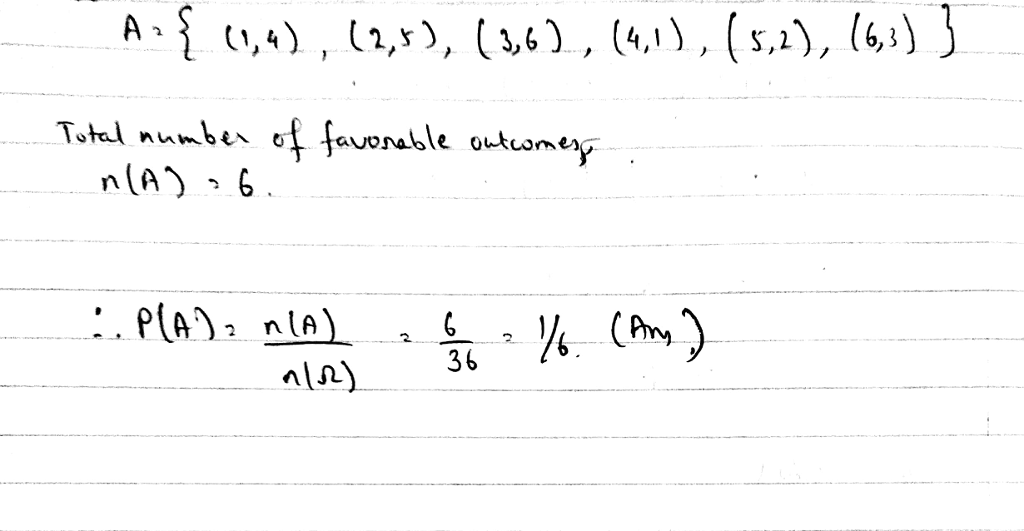#### Earn Coins

Coins can be redeemed for fabulous gifts.

Similar Homework Help Questions
• ### Three fair six-sided dice are rolled. a) What is the probability of seeing {1, 3, 6}?...

Three fair six-sided dice are rolled. a) What is the probability of seeing {1, 3, 6}? b) What is the probability of seeing {1, 4, 4}? c) What is the probability of seeing {2, 2, 2} ? d) What is the probability of seeing at least one 6? e) What is the probability that the sum of all three dice is 16? f) What is the probability of seeing exactly two even numbers?

• ### Three six-faced fair dice are rolled. What is the probability that exactly two of the dice...

Three six-faced fair dice are rolled. What is the probability that exactly two of the dice have the face value of 6?

• ### Three six-sided fair dice are rolled. The six sides are numbered 1,2,3,4,5,6. Let A be the...

Three six-sided fair dice are rolled. The six sides are numbered 1,2,3,4,5,6. Let A be the event that the first die shows an even number, let B be the event that the second die shows an even number, and let C be the event that the third die shows an even number. Express each of the following events in terms of the named events described above: 1) the event that all three dice show even numbers 2) the event that...

• ### A six sided die is rolled three times

A six sided die is rolled three times. List possible outcomes and find the probability of rolling exactly one 6.

• ### What is the probability that a fair six-sided die rolled five times comes up 6 exactly...

What is the probability that a fair six-sided die rolled five times comes up 6 exactly once?

• ### 1.) Suppose you roll two fair six-sided dice. What is the probabilty that I rolled a...

1.) Suppose you roll two fair six-sided dice. What is the probabilty that I rolled a total of 5? 2.) Suppose you roll two fair six-sided die and I announce that the sun of the two die is 6 or less. What is the probabilty that you rolled a total of 5?

• ### 7. (3 points) Given a fair 6-sided die. Each time the die is rolled, the probabilities...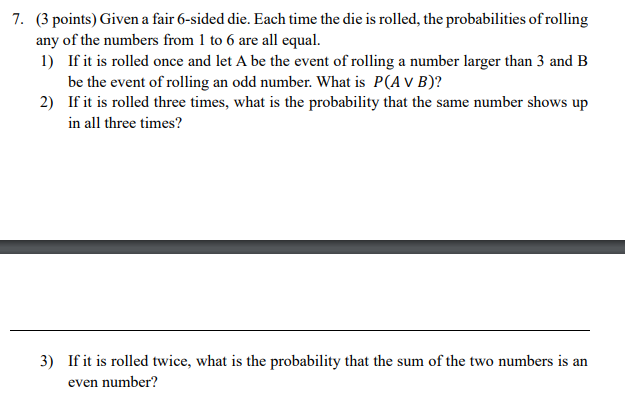7. (3 points) Given a fair 6-sided die. Each time the die is rolled, the probabilities of rolling any of the numbers from 1 to 6 are all equal. 1) If it is rolled once and let A be the event of rolling a number larger than 3 and B be the event of rolling an odd number. What is P(AV B)? 2) If it is rolled three times, what is the probability that the same number shows up in...

• ### In this experiment, both a fair four-sided die and a fair six-sided die are rolled (these...

In this experiment, both a fair four-sided die and a fair six-sided die are rolled (these dice both have the numbers most people would expect on them). Let Z be a random variable that represents the absolute value of their difference. For instance, if a 4 and a 1 are rolled, the corresponding value of Z is 3. (a) What is the pmf of Z? (b) Draw a graph of the cdf of Z

• ### 1. A blue fair 6-sided dice and a red fair 6-sided dice are rolled at the...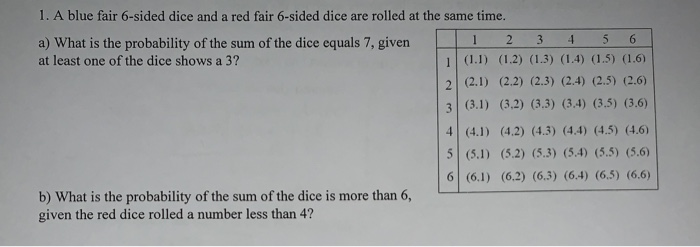1. A blue fair 6-sided dice and a red fair 6-sided dice are rolled at the same time. a) What is the probability of the sum of the dice equals 7, given 1 2 3 4 5 6 at least one of the dice shows a 3? 1 (1.1) (1.2) (1.3) (1.4) (1.5) (1.6) 2 (2.1) (2.2) (2.3) (2.4) (2.5) (2.6) (3.1) (3.2) (3.3) (3.4) (3.5) (3.6) (4.1) (4.2) (4.3) (4.4) (4.5) (4.6) 5 (5.1) (5.2) (5.3) (5.4) (5.5) (5.6)...

• ### A six-sided dice is rolled twice. Find the probability that the larger of the two rolls...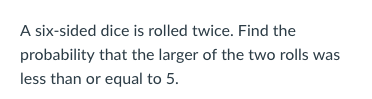A six-sided dice is rolled twice. Find the probability that the larger of the two rolls was less than or equal to5 A fair coin is flipped 3 times. Find the probability that exactly 1 of the flips will turn up as heads.